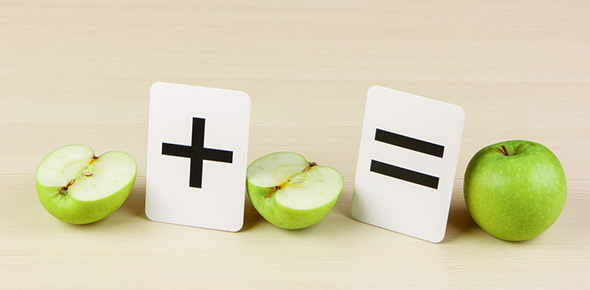# Mathematics II ( Fraction )

10 QuestionsSettingsSubject: MATHEMATICS (fraction)

Related Topics
• 1.
fraction represents a part of a whole or, more generally, any number of equal parts.
• A.

True

• B.

False

• 2.
Based on your own explanation. what is fraction?
• 3.
Fractions were ___________  of integers
• 4.
_________ is the term of a fraction, usually above the line,that indicates the number of equal parts that are to beadded together; the dividend placed over a divisor
• 5.
___________ is a term of a fraction, usually written underthe line.
• 6.
4 / 8 = ?
• A.

1

• B.

2

• C.

3

• D.

4

• 7.
2 / 10 = ?
• A.

4

• B.

5

• C.

6

• D.

7

• 8.
3 / 9 = ?
• A.

A. 1

• B.

B. 2

• C.

C. 3

• D.

D. 4

• 9.
is that a 5/10?
• A.

True

• B.

False

• 10.
• A.
• B.